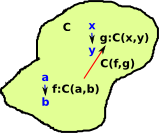# Maths - Functor - Homset

## Hom Functors

 Given a category with objects such as: a,b...x,y f:C(a,b) represents all the morphisms from a to b. g:C(x,y) represents all the morphisms from x to y. We can now take this up one level. A hom functor C(f,g) represents all the functors from f to g.We require this hom functor to interact nicely with the hom sets so the diagram on the left needs to commute.

This gives rise to a bi-functor to the category of sets:

Cop×C->Set

A bifunctor is a functor in two arguments, it could be written H(Cop,C)->Set . That is, it is contravarient in the first argument and covarient in the second).

## Next steps

Hom sets are used in the page about the Yonada Lemma to relate structures at different levels of abstraction.

#### Other Related Pages

• Hom sets can be objects of a category which is the exponential object discussed on page here.
• The page here discuses homsets from a set theory perspective.
• Other related pages are:

Where I can, I have put links to Amazon for books that are relevant to the subject, click on the appropriate country flag to get more details of the book or to buy it from them.The Princeton Companion to Mathematics - This is a big book that attempts to give a wide overview of the whole of mathematics, inevitably there are many things missing, but it gives a good insight into the history, concepts, branches, theorems and wider perspective of mathematics. It is well written and, if you are interested in maths, this is the type of book where you can open a page at random and find something interesting to read. To some extent it can be used as a reference book, although it doesn't have tables of formula for trig functions and so on, but where it is most useful is when you want to read about various topics to find out which topics are interesting and relevant to you.